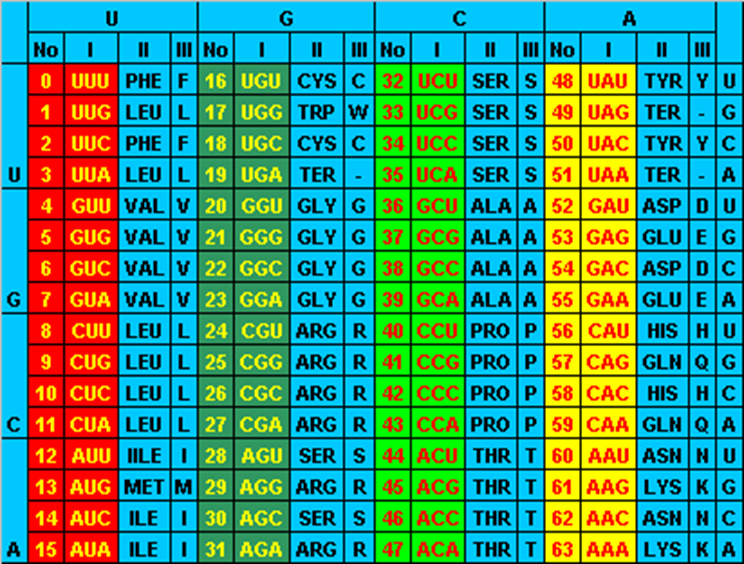# Genome Algebra Research

The genetic code algebras and its extension to genes and genomes involve several algebraic structures, such as: Boolean algebras [1,2], modular algebras , vector spaces and Galois fields . Each algebraic structure provides a different approach for the understanding of the gene and genome architectures, as well as, the mutational and the molecular evolutionary process. For example, the Boolean algebra provides the way to understand the operational logic of the mutational process [1,2], either on the four-letter alphabet of the DNA molecules or on the binary alphabet used by modern computers. The genetic code vector space on the Galois field of four DNA bases revealed that the quantitative relationships between codons determine a genetic code architecture mathematically equivalent to a cube inserted in the three-dimensional space . The genetic code algebras are founded on the quantitative relationships given between DNA bases in the codons.

The genetic code is the code of the genetic communication/information system (GCS) . Most of the message in the GCS are written in the four DNA bases alphabet. These “letters” are the DNA bases: adenine, guanine, cytosine, and thymine, usually denoted A, G, C, and T respectively (in an RNA molecule, T is changed to U, uracil). They are paired according to the following rule (Watson – Crick base pairings): G:C, A:T. That is, base G is the complementary base of C, and A is the complementary base of T (or U) in the DNA (or in the RNA) molecule and vice-versa. The standard genetic code table (RNA codon table) is formed by 64 codons.

In superior organisms there is also evidence supporting an epigenome communication system (ECS), which is an extension of the GCS . The alphabet of the ECS is an extension of the GCS, which includes methylated cytosine (mainly) and adenine. Genetic code algebras and their extesions to genes and genomes have been already developed.

The genetic code tableColumns of the genetic code table are not at random. It is well known that there is an association between second-position base and hydrophobicity; in which the amino acid that have U at the second position of their codon are hydrophobic: {I, L, M, F}, whereas those that have A at the second position are hydrophilic (polar amino acids): {D, E, H, N, K, Q, Y}. This was highlighted by Crick when he proposed his famous hypothesis about the accidental frozen code . Epstein  pointed out that “related” amino acids have to some extent related codons and Crick  considered that the amino acid in the genetic code table does not seem to be allocated in a totally random way. So it is natural to think that some partial order in the codons set should reflect the physico-chemical properties of amino acid [9,10].

### A Short Introduction to Algebraic Taxonomy on Genes Regions

a-short-introduction-to-algebraic-taxonomy-on-genes-regions A Short Introduction to Algebraic Taxonomy on Genes Regions¶ Herein, we show a short and simply introduction to algebraic taxonomy of genomic/genes regions based on the nalysis of DNA mutational events on DNA Multiple Sequence Alignment (MSA) by means of automorphisms between pairwise DNA sequences algebraically represented as Abelian finite group. Overview¶ GenomAutomorphism is […]

### The Binary Alphabet of DNA

On the DNA Computer Binary Code In any finite set we can define a partial order, a binary operation in different ways. But here, a partial order is defined in the set of four DNA bases in such a manner that a Boolean lattice structure is obtained. A Boolean lattice is an algebraic structure that […]

### The genetic-code vector space B^3 over the Galois field GF(5)

The $mathbb{Z_5}$-vector space $mathfrak{B}$3 over the field $(mathbb{Z_5}, +, .)$ 1. Background This is a formal introduction to the genetic code $mathbb{Z_5}$-vector space $mathfrak{B}^3$ over the field $(mathbb{Z_5}, +, .)$. This mathematical model is defined based on the physicochemical properties of DNA bases (see previous post). This introduction can be complemented with a Wolfram Computable […]

### Group operations on the set of five DNA bases

An introduction to the groups defined on five DNA bases General biochemical background. The genetic information on how to build proteins able to perform different biological/biochemical functions is encoded in the DNA sequence. Code-words of three letters/bases, called triplets or codons, are used to encode the information that will be used to synthesize proteins. Every […]

### References

1. Sanchez R, Morgado E, Grau R. The genetic code Boolean lattice. MATCH Commun Math Comput Chem, 2004, 52:29–46
2. Sánchez R, Morgado E, Grau R. A genetic code Boolean structure. I. The meaning of Boolean deductions. Bull Math Biol, 2005, 67:1–14
3. Sanchez R, Morgado E, Grau R. Gene algebra from a genetic code algebraic structure. J Math Biol, 2005, 51:431–57
4. Sánchez R, Perfetti LA, Grau R, Morales ERM. A New DNA Sequences Vector Space on a Genetic Code Galois Field. MATCH Commun Math Comput Chem, 2005, 54:3–28.
5. Sanchez R, Grau R. Bull Math Biol, 2005, 67:1017–29
6. Sanchez R, Mackenzie SA. Information Thermodynamics of Cytosine DNA Methylation. PLoS One, 2016, 11:e0150427.
7. Crick FHC. The Origin of the Genetic Code. J Mol Biol, 1968, 38:367–79.
8. Epstein, C.J. Role of the amino-acid “code” and of selection for conformation in the evolution of proteins. Nature, 1966, 210, 25-28.
9. Lehmann, J. J. Theor. Biol. 2000, 202, 129-144.
10. Robin D, Knight RD, Freeland SJ, Landweber LF. Selection, history and chemistry: the three faces of the genetic code. Trends Biochem Sci. 1999, 24: 241-247